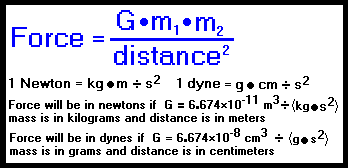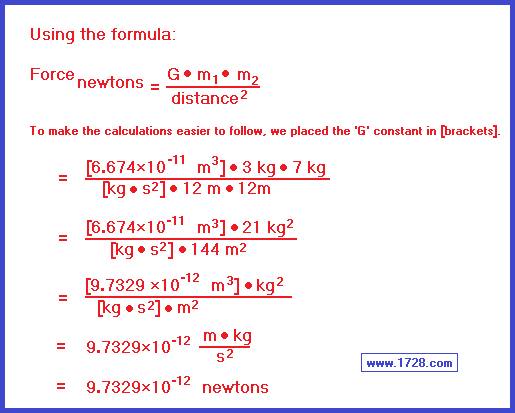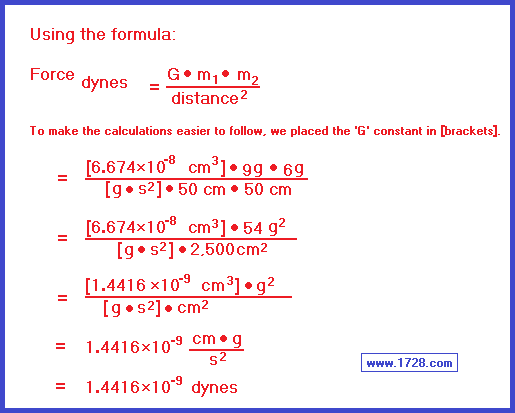Gravitational Force CalculatorThe above equation is Newton's Law of Gravitation formulated by British physicist, mathematician and astronomer Sir Isaac Newton (1642-1727).

The following 2 examples will help familiarize yourself with the formulas and with this calculator.
After trying both methods, you will agree that using the calculator is much easier than using the formula.

 1) What is the gravitational force between one object of 3 kilograms of mass and another object of 7 kilograms of mass that are 12 meters apart? Using the calculator: Click the 'Meters & Kilograms' button, then enter the numbers, click 'Calculate' and the answer is 9.7329 x 10-12 newtons. or you can use this method:2) Calculate the force between one object of 9 grams of mass and another of 6 grams that are 50 centimeters meters apart. Using the calculator: Click the 'Centimeters & Grams' button, enter the numbers, click 'Calculate' and the answer is 1.4416 x 10-9 dynes. or you can use this method:or
>>>>>
>>>>>
>>>>>

>>>>>

Significant Figures >>>
Numbers are displayed in scientific notation with the amount of significant figures you specify. For easier readability, numbers between .001 and 1,000 will not be in scientific notation but will still have the same precision.
You may change the number of significant figures displayed by changing the number in the box above.
Most browsers, will display the answers properly but if you are seeing no answers at all, enter a zero in the box above, which will eliminate all formatting but at least you will see the answers.